Try NerdPal! Our new app on iOS and Android

# Find the limit of $\frac{t^2+3t-10}{t^3-2t^2+t-2}$ as $t$ approaches $2$

## Related Videos

Go!
Go!
1
2
3
4
5
6
7
8
9
0
a
b
c
d
f
g
m
n
u
v
w
x
y
z
.
(◻)
+
-
×
◻/◻
/
÷
2

e
π
ln
log
log
lim
d/dx
Dx
|◻|
θ
=
>
<
>=
<=
sin
cos
tan
cot
sec
csc

asin
acos
atan
acot
asec
acsc

sinh
cosh
tanh
coth
sech
csch

asinh
acosh
atanh
acoth
asech
acsch
Solving: $\lim_{t\to2}\left(\frac{t^2+3t-10}{t^3-2t^2+t-2}\right)$

### Videos### Factoring a trinomials to find the zeros of a function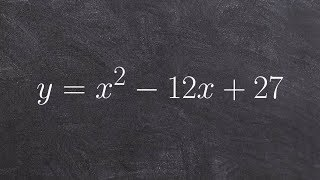### Find the zeros by factoring a quadratic factoring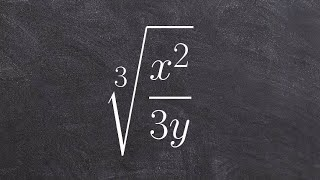### Algebra 2 - How to divide the cube root of a rational expression by rationalizing the denominator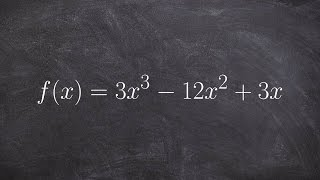### Solve for the Zeros Using Quadratic Formula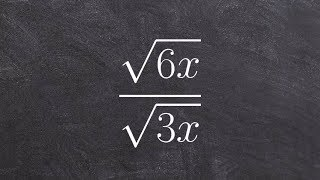### Algebra 2 - Simplifying a radical expression by dividing and not rationalizing the denominator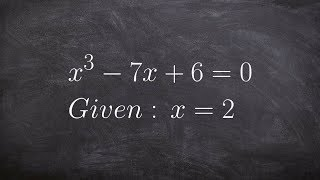SnapXam A2

### beta Got another answer? Verify it!

Go!
1
2
3
4
5
6
7
8
9
0
a
b
c
d
f
g
m
n
u
v
w
x
y
z
.
(◻)
+
-
×
◻/◻
/
÷
2

e
π
ln
log
log
lim
d/dx
Dx
|◻|
θ
=
>
<
>=
<=
sin
cos
tan
cot
sec
csc

asin
acos
atan
acot
asec
acsc

sinh
cosh
tanh
coth
sech
csch

asinh
acosh
atanh
acoth
asech
acsch

$\lim_{t\to2}\left(\frac{t^2+3t-10}{t^3-2t^2+t-2}\right)$